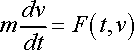# Question on Function Notation

Artimodes
Hello everyone,

I'm a newcomer to this forum! I've been having particular difficulty lately with understanding function notation in higher level mathematics. I felt like it was a general post so I posted in the general math section. I've finished calculus 3 and am going to take engineering math (basically ODE and applications) next spring.

It is my understanding that for a three dimensional function, for example an elliptic paraboloid, it's written like:

f(x,y)= z =x2+y2

However, after looking at Paul's Online Notes on ODE here, he starts to describe Newton's Second Law as a differential equation. I knew that, but his notation threw me off:Is force in this case a three dimensional function, due to force being a function of the derivative of velocity while velocity is a function of time? Just confused.

Thanks for any help